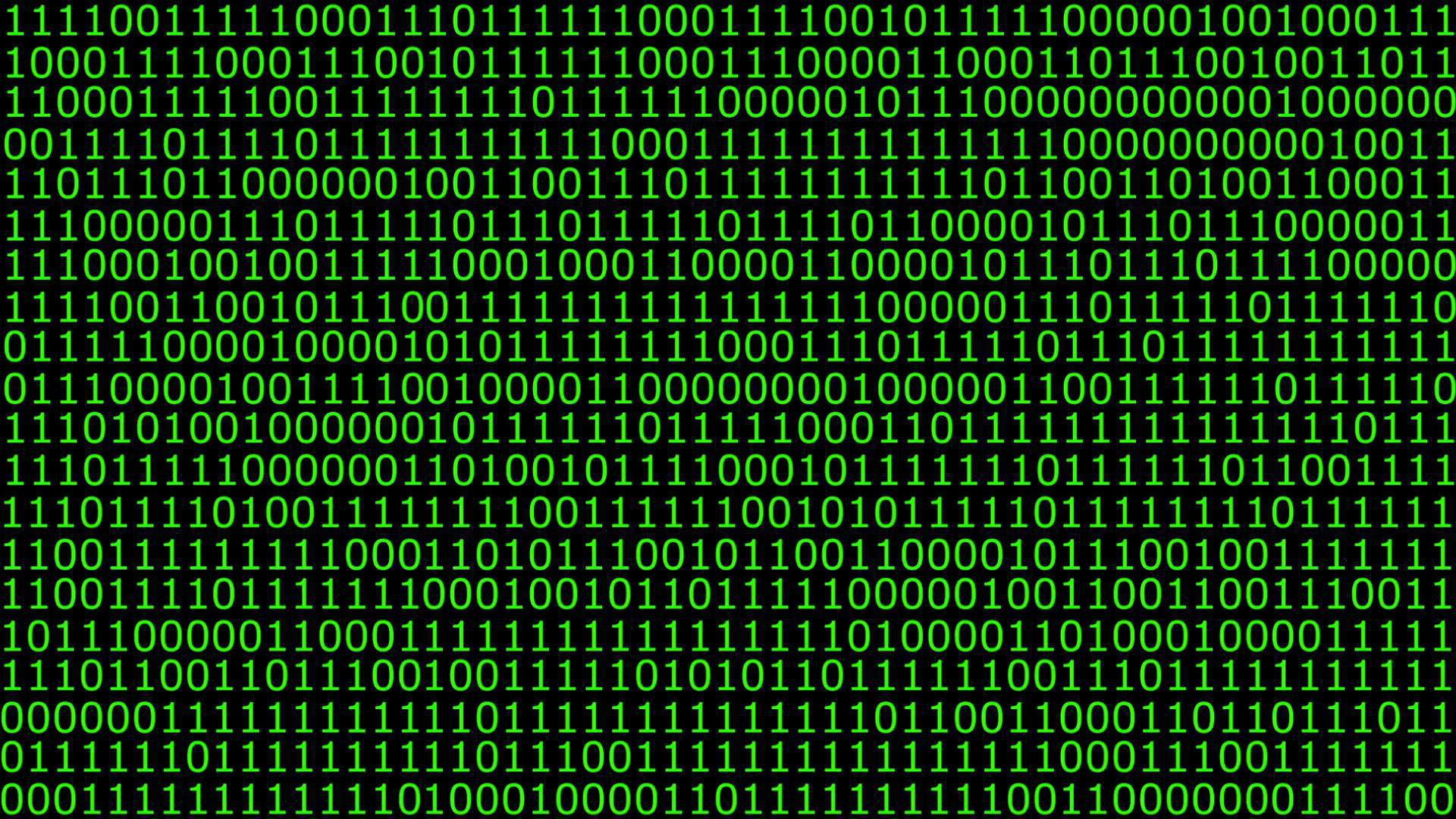# Coding Theory - Linear CodesThis will concern mostly the section of linear codes in the course of Coding Theory & Data Compression at Cardiff University. It is expected the reader knows about some sections of coding theory, there isn’t background reading on this blog… yet­ƒòú. ___ Things to know to start:

• The alphabet we will be using is the set $$F_q$$ where $$q$$ is prime.
• We will regard the vector space $$V(n,q)$$ as the set of words $$(F_q)^n$$, a vector in $$V(n,q)$$ denoted $$(x_1,x_2,…,x_n)$$ will be written as $$x_1 x_2 … x_n$$.
• A linear code $$C$$ is just a subset of the space $$V(n,q)$$
• This code will a linear code in itself if and only if it is a vector space under the same operations as $$V(n,q)$$.
• A binary code will be linear if and only if the sum of any two words is itself a word in the original set. i.e. $$\forall x,y \in C \quad (x + y) \in C$$

Note: 1) A $$q-$$ary code $$[n,k,d]$$ is also a $$q-$$ary code $$(n,q^k,d)$$ code (by a theorem on basis of subspaces). This is not two way, $$[n,k,d] \Rightarrow (n,q^k,d)$$ but $$(n,q^k,d) \not\Rightarrow [n,k,d]$$. 2) The $$\bf{0}$$ vector is automatically in any linear code. 3) Linear Codes may be referred to as Group Codes in some texts. 4) The terms “word” and “vector” are synonyms in the context of a linear code. ___

The weight, $$w(\bf{x})$$, of a word in $$V(n,q)$$ is defined to be the number of non-zero entries in the word: $$\bf{x}=111010$$ has $$w(\bf{x})=2)$$.

One of the most useful properties of a linear code is that $$d(C)$$, its minimum distance, is equal to the smallest of the wights of its codewords: $$d(C)= min(w(\bf{x})) \quad \forall \bf{x} \in C$$.